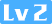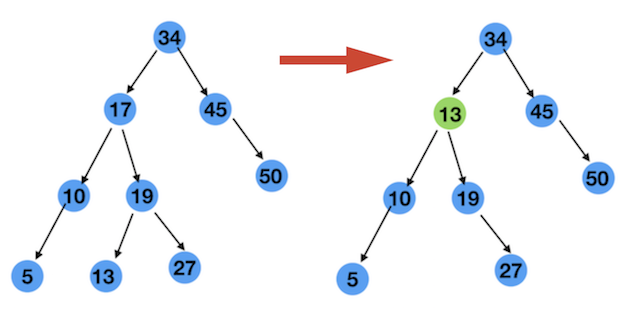7 二分搜索树的原理与Java源码实现九路222 0 0

## 1 折半查找法

int[] arr = new int[]{1,3,4,6,8,9};

java实现代码如下：

    private static int binarySearch(int[] data, int target) {
int l = 0;
int r = data.length - 1;

while (l <= r) {
//int mid = (l + r) / 2;
//这句代码理论上是没有问题的，但是是有bug的
//如果因为 l + r 会超过整数的最大值，就会溢出
//所以换成下面的写法，最小边界，加上差的一半，就是中间索引

//最小边界，加上差的一半，就是中间值
int mid = l + (r - l) / 2;

if (data[mid] > target) { //如果中间的值比target大,r向右移动。
r = mid - 1;
} else if (data[mid] < target) { //如果中间的值比target小，l向左移动
l = mid + 1;
} else {
return mid; //如果中间的值与target相等，就返回下标
}
}

//没有找到就返回-1
return -1;
}

 public static void main(String[] args) {
int[] data = new int[]{1,3,4,6,8,9};
System.out.println(binarySearch(data, 6));
}

3

## 2 二分搜索树定义

### 2.0 二叉搜索树的定义和节点的定义

    //key必须继承Comparable，可以比较大小的
public class QBST<K extends Comparable<K>, V> {
...
}

   //QNode是作为QBST的内部类的。后面会有完整的源码
class QNode {
//key,也相当于上图中的数字，只不过不一定是数字
//只要能比较大小就行了。这里的key，是继承Comparable的
K key;

//节点中的value
V value;

//左子树
QNode left;

//右子树
QNode right;

//根据key,value构造一个节点
QNode(K key, V value) {
this.key = key;
this.value = value;
this.left = null;
this.right = null;
}

//根据一个节点，构造另一个新节点
QNode(QNode node){
this.key = node.key;
this.value = node.value;
this.left = node.left;
this.right = node.right;
}
}

/**
* 二分搜索树，也叫二分查找树
*/
public class QBST<K extends Comparable<K>, V> {
class QNode {
K key;
V value;
QNode left;
QNode right;

QNode(K key, V value) {
this.key = key;
this.value = value;
this.left = null;
this.right = null;
}

QNode(QNode node){
this.key = node.key;
this.value = node.value;
this.left = node.left;
this.right = node.right;
}
}

//树的根
private QNode root;
//树中节点的个数
private int count;

//构造一棵空的二分搜索树
public QBST() {
root = null;
count = 0;
}

//返回二分搜索树中的个数
public int size() {
return count;
}

//树是否为空
public boolean isEmpty() {
return count == 0;
}

}

### 2.1 二叉搜索树的插入

1 如果这棵树为空，新建一个节点，作为根 2 如果要插入的key比根节点大，就插入到右子树中 3 如果要插入的key比根节点小，就插入到左子树中 4 如果要插入的key和根节点相等，就更新当前节点的value 代码如下：

 public void insert(K key, V value) {
root = insert(root, key, value);
}

// 向以node为根的二叉搜索树中，插入节点(key,value)
// 返回插入新节点后的二叉搜索树的根
private QNode insert(QNode node, K key, V value) {
//查检条件
checkNotNull(key,"key is null");

//如果node为空,直接new一个节点返回
if (node == null) {
count++;
return new QNode(key, value);
}

//如果key比根节点大，插入到node的右子树中
if (key.compareTo(node.key) == 1) {
node.right = insert(node.right, key, value);

//如果key比根节点小，插入到node的左子树中
} else if (key.compareTo(node.key) == -1) {
node.left = insert(node.left, key, value);

//如果key和根节点相等，更新根节点的value
} else {
node.value = value;
}

//返回根
return node;
}

### 2.2 二叉搜索树的查找

   //搜索key结果的value
public V search(K key){
return search(root,key);
}

// 向以node为根的二叉搜索树中，以key为键，返回V
private V search(QNode node,K key){
checkNotNull(key,"key is null");

//如果当前节点为null,返回null
if(node == null){
return null;
}

//如果key比根节点的key大，在右子树中查找
if(key.compareTo(node.key) == 1){
return search(node.right,key);

//如果key比根节点的key小，在左子树中查找
}else if(key.compareTo(node.key) == -1){
return search(node.left,key);

//如果key与根节点的key值相等，就返回节点的value值
}else {
return node.value;
}
}

### 2.3 二叉搜索树的遍历

    // 前序遍历 O(n)
public void preOrder(){
//后序遍历以root为根的二叉搜索树
preOrder(root);
}

private void preOrder(QNode node){
if(node != null){
//先遍历根节点
System.out.println(node.key);//这里的访问只是打印
//前序遍历左子树
preOrder(node.left);
//后序遍历右子树
preOrder(node.right);
}
}

// 中序遍历 O(n)
public void middleOrder(){
middleOrder(root);
}

private void middleOrder(QNode node){
if(node != null){
middleOrder(node.left);
System.out.println(node.key);
middleOrder(node.right);
}
}

// 后序遍历 O(n)
public void postOrder(){
postOrder(root);
}

private void postOrder(QNode node){
if(node != null){
postOrder(node.left);
postOrder(node.right);
System.out.println(node.key);
}
}


   // 层序遍历，也叫做广度优先遍历
public void levelOrder(){
if (root == null){
return;
}

while (!q.isEmpty()){
QNode node = q.removeFirst();
System.out.println("节点的值是：" + node.key);

if(node.left != null){
}
if(node.right != null){
}

}
}


### 2.4 二叉搜索树的删除

1. 删除二叉搜索树中最小的节点
2. 删除二叉搜索树中最大的节点
3. 查找二叉搜索树中最小的节点
4. 查找二叉搜索树中最大的节点

1. 在一个二叉搜索树中，最小的节点一定是最左边的节点，也就是图中的节点 3
2. 在一个二叉搜索树中，最大的节点一定是最右边的节点，也就是图中的节点 18

1 先来实现查找二叉搜索树中最小的节点 如下代码

    //查找一棵树中最小的节点，返回 K
public K minimum(){
checkNotNull(root,"the tree is empty");

//在以根为root的二叉搜索树中返回最小节点的键值
QNode minNode = minimum(root);

//返回最小节点的键值
return minNode.key;
}

// 在以node为根的二叉搜索树中，返回最小键值的节点
private QNode minimum(QNode node){
//如果node.left == null,说明当前node节点就是最小的节点
//返回当前节点node
if(node.left == null){
return node;
}

//如果当前节点不是最小的节点
//继承往左子树中查找
return minimum(node.left);
}


public K maximum(){
checkNotNull(root,"the tree is empty");
QNode maxNode = maximum(root);
return maxNode.key;
}

// 在以node为根的二叉搜索树中，返回最大键值的节点
private QNode maximum(QNode node){
if(node.right == null){
return node;
}

return maximum(node.right);
}

3 实现删除二叉搜索树中最小的节点 一直往左孩子中删除，当某一个节点node没有左孩子时，说明当前节点就是最小节点 这时候分两种情况

1. 当前节点有右孩子 如果是这种情况，直接把右孩子返回，作为当前节点
2. 当前节点没有右孩子 如果是这种情况，直接返回null。此时返回右孩子也行，因为右孩子也是null

    // 删除二叉搜索树中最小的节点
public void removeMin(){
if(root != null){
root = removeMin(root);
}
}

// 删除掉以node为根的二分搜索树中的最小的节点
// 返回删除节点后新的二分搜索树的根
private QNode removeMin(QNode node){
//如果当前当前没有左孩子，则当前节点就是最小节点
if(node.left == null){
//保存当前节点的右孩子，这句代码把上面两种情况都包含了
QNode rightNode = node.right;
node = null;    //释放当前节点
count--;        //记得数量要减1
return rightNode;//返回右孩子，有可能为空或者不为空
}

//递归调用删除以当前节点的左孩子为根的二叉搜索中最小的节点
node.left = removeMin(node.left);

//别忘了返回当前节点
return node;
}

   // 删除二叉搜索树中最大的节点
public void removeMax(){
if(root != null){
root = removeMax(root);
}
}

// 删除掉以node为根的二分搜索树中的最大的节点
// 返回删除节点后新的二分搜索树的根
private QNode removeMax(QNode node){
if(node.right == null){
QNode leftNode = node.left;
count--;
node = null;

return leftNode;
}

node.right = removeMax(node.right);
return node;
}

1. node 没有孩子
2. node 只有一个孩子
3. node 有两个孩子

1. node节点有一个左孩子
2. node节点有一个右孩子

1. 17大于它的左孩子10
2. 17小于它的右孩子19

1. 大于 10 的节点，只要在 17 的右子树 也就是以 19 为根节点的树中找不就行了吗 因为17的右子树中所有的节点都比 17 大
2. 小于 19 的节点，只要在以 19 为根的树中找左孩子不就得了吗 经过上面的分析，这样的节点就是 13 啊，将17删除 ，把13放到17的位置 ，如图10和13两个节点都满足条件，所以我们可以得出结论

s 可以叫作 d 的后继 s.right = deledeMin(d->right) s.left = d.left; 删除 d, s 是新的子树的根

  public void remove(K key) {
root = remove(root, key);
}

// 删除掉以node为根的二分搜索树中键值为key的节点
// 返回删除节点后新的二分搜索树的根
// O(logN)
private QNode remove(QNode node, K key) {
//如果树为null,返回null
if (node == null) {
return null;
}

//想要删除某个节点，必须先要找到这个节点
//所以下面的代码包含了查找

if (key.compareTo(node.key) == -1) {//如果key小于根节点的key

//到node的左子树查找并删除键值为key的节点
node.left = remove(node.left, key);

//返回删除节点后新的二分搜索树的根
return node;

} else if (key.compareTo(node.key) == 1) {//如果key大于根节点的key

//到node的右子树查找并删除键值为key的节点
node.right = remove(node.right, key);

//返回删除节点后新的二分搜索树的根
return node;
} else { //key == node.key,也就是找到了这个节点

//当前节点的左孩子为null
if (node.left == null) {
//保存右孩子节点
QNode rightNode = node.right;
//个数减1
count--;

//删除
node = null;

//右节点作为新的根
return rightNode;
}

//当前节点的右孩子为null
if (node.right == null) {
//保存左孩子的节点
QNode leftNode = node.left;
//个数减1
count--;

//删除
node = null;

//左节点作为新的根
return leftNode;
}

//上面的情况也包括了左右两个孩子都是null
//这样的情况就走第一种，node.left==null的条件中。也满足

//下面是 node.left != null && node.right != null的情况

//找到右子树中最小节点
QNode min = minimum(node.right);

//用最小节点新建一个节点,因为等会要删除最小的节点，所以这里我们要新建一个最小节点
QNode s = new QNode(min);

//s的右孩子，就是删除node右子树中最小节点返回的根
s.right = removeMin(node.right);

//s的左孩子，就是删除节点的左孩子
s.left = node.left;

//返回新的根
return s;
}
}


/**
* 二分搜索树，也叫二分查找树
*/
public class QBST<K extends Comparable<K>, V> {
class QNode {
K key;
V value;
QNode left;
QNode right;

QNode(K key, V value) {
this.key = key;
this.value = value;
this.left = null;
this.right = null;
}

QNode(QNode node) {
this.key = node.key;
this.value = node.value;
this.left = node.left;
this.right = node.right;
}
}

private QNode root;
private int count;

public QBST() {
root = null;
count = 0;
}

public int size() {
return count;
}

public boolean isEmpty() {
return count == 0;
}

public void insert(K key, V value) {
root = insert(root, key, value);
}

// 向以node为根的二叉搜索树中，插入节点(key,value)
// 返回插入新节点后的二叉搜索树的根
private QNode insert(QNode node, K key, V value) {
checkNotNull(key, "key is null");

if (node == null) {
count++;
return new QNode(key, value);
}

if (key.compareTo(node.key) == 1) {
node.right = insert(node.right, key, value);
} else if (key.compareTo(node.key) == -1) {
node.left = insert(node.left, key, value);
} else {
node.value = value;
}

return node;
}

public boolean contain(K key) {
return contain(root, key);
}

// 向以node为根的二叉搜索树中，查找是否包含key的节点
private boolean contain(QNode node, K key) {
checkNotNull(key, "key is null");

if (node == null) {
return false;
}

if (key.compareTo(node.key) == 1) {
return contain(node.right, key);
} else if (key.compareTo(node.key) == -1) {
return contain(node.left.key);
} else {
return true;
}
}

public V search(K key) {
return search(root, key);
}

// 向以node为根的二叉搜索树中，
private V search(QNode node, K key) {
checkNotNull(key, "key is null");

if (node == null) {
return null;
}

if (key.compareTo(node.key) == 1) {
return search(node.right, key);
} else if (key.compareTo(node.key) == -1) {
return search(node.left, key);
} else {
return node.value;
}
}

// 前序遍历 O(n)
public void preOrder() {
preOrder(root);
}

private void preOrder(QNode node) {
if (node != null) {
System.out.println(node.key);
preOrder(node.left);
preOrder(node.right);
}
}

// 中序遍历 O(n)
public void middleOrder() {
middleOrder(root);
}

private void middleOrder(QNode node) {
if (node != null) {
middleOrder(node.left);
System.out.println(node.key);
middleOrder(node.right);
}
}

// 后序遍历 O(n)
public void postOrder() {
postOrder(root);
}

private void postOrder(QNode node) {
if (node != null) {
postOrder(node.left);
postOrder(node.right);
System.out.println(node.key);
}
}

// 层序遍历，也叫做广度优先遍历
public void levelOrder() {
if (root == null) {
return;
}

while (!queue.isEmpty()) {
QNode node = queue.removeLast();
System.out.println(node.key);
}
}

public void destroy() {
destroy(root);
}

// 销毁操作就是后序遍历的一次应用
private void destroy(QNode node) {
if (node != null) {
destroy(node.left);
destroy(node.right);

node = null;
count--;
}
}

public K minimum() {
checkNotNull(root, "the tree is empty");
QNode minNode = minimum(root);
return minNode.key;
}

// 在以node为根的二叉搜索树中，返回最小键值的节点
private QNode minimum(QNode node) {
if (node.left == null) {
return node;
}

return minimum(node.left);
}

public K maximum() {
checkNotNull(root, "the tree is empty");
QNode maxNode = maximum(root);
return maxNode.key;
}

// 在以node为根的二叉搜索树中，返回最大键值的节点
private QNode maximum(QNode node) {
if (node.right == null) {
return node;
}

return maximum(node.right);
}

// 删除二叉搜索树中最小的节点
public void removeMin() {
if (root != null) {
root = removeMin(root);
}
}

// 删除掉以node为根的二分搜索树中的最小的节点
// 返回删除节点后新的二分搜索树的根
private QNode removeMin(QNode node) {
if (node.left == null) {
QNode rightNode = node.right;
node = null;
count--;
return rightNode;
}

node.left = removeMin(node.left);
return node;
}

// 删除二叉搜索树中最大的节点
public void removeMax() {
if (root != null) {
root = removeMax(root);
}
}

// 删除掉以node为根的二分搜索树中的最大的节点
// 返回删除节点后新的二分搜索树的根
private QNode removeMax(QNode node) {
if (node.right == null) {
QNode leftNode = node.left;
count--;
node = null;

return leftNode;
}

node.right = removeMax(node.right);
return node;
}

public void remove(K key) {
root = remove(root, key);
}

// 删除掉以node为根的二分搜索树中键值为key的节点
// 返回删除节点后新的二分搜索树的根
// O(logN)
private QNode remove(QNode node, K key) {
//如果树为null,返回null
if (node == null) {
return null;
}

//想要删除某个节点，必须先要找到这个节点
//所以下面的代码包含了查找

if (key.compareTo(node.key) == -1) {//如果key小于根节点的key

//到node的左子树查找并删除键值为key的节点
node.left = remove(node.left, key);

//返回删除节点后新的二分搜索树的根
return node;

} else if (key.compareTo(node.key) == 1) {//如果key大于根节点的key

//到node的右子树查找并删除键值为key的节点
node.right = remove(node.right, key);

//返回删除节点后新的二分搜索树的根
return node;
} else { //key == node.key,也就是找到了这个节点

//当前节点的左孩子为null
if (node.left == null) {
//保存右孩子节点
QNode rightNode = node.right;
//个数减1
count--;

//删除
node = null;

//右节点作为新的根
return rightNode;
}

//当前节点的右孩子为null
if (node.right == null) {
//保存左孩子的节点
QNode leftNode = node.left;
//个数减1
count--;

//删除
node = null;

//左节点作为新的根
return leftNode;
}

//上面的情况也包括了左右两个孩子都是null
//这样的情况就走第一种，node.left==null的条件中。也满足

//下面是 node.left != null && node.right != null的情况

//找到右子树中最小节点
QNode min = minimum(node.right);

//用最小节点新建一个节点,因为等会要删除最小的节点，所以这里我们要新建一个最小节点
QNode s = new QNode(min);

//s的右孩子，就是删除node右子树中最小节点返回的根
s.right = removeMin(node.right);

//s的左孩子，就是删除节点的左孩子
s.left = node.left;

//返回新的根
return s;
}
}

private <E> void checkNotNull(E e, String message) {
if (e == null) {
throw new IllegalArgumentException(message);
}
}

}